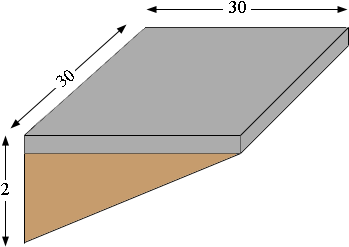SEARCH HOMEMath Central Quandaries & QueriesQuestion from Rose: We are going to be pouring a driveway and it is needing a lot of fill. I can't seem to figure this out. Measurements are 30 ft x 30 ft and it goes from 2' deep to 4" (which is what I need overall as it will be 4" of concrete for the driveway. Please HELP Rose in GA.Hi Rose,

Here is my "not-to-scale" sketch of your driveway.The volume of fill required is the area of the triangular cross-section, shaded brown, times the length of 30 feet. You probably want the volume in cubic yards so first convert all the measurements to yards. The surface is 30/3 = 10 yards by 10 yards and the height of the triangular fill cross-section is 24-3 = 21 inches which is 21/36 = 7/12 yards. Hence the area of the triangular cross-section is

1/2 × base × height = 1/2 ×10 × 7/12 square yards.

Thus the volume of fill required is

1/2 ×10 × 7/12 × 10 = 29.1 cubic yards.

I hope this helps,
PennyMath Central is supported by the University of Regina and The Pacific Institute for the Mathematical Sciences.